Скачать презентацию Modern Atomic Theory a k a the electron

c50437f3dc99e3363cf78f3a8cd69fc5.ppt

• Количество слайдов: 54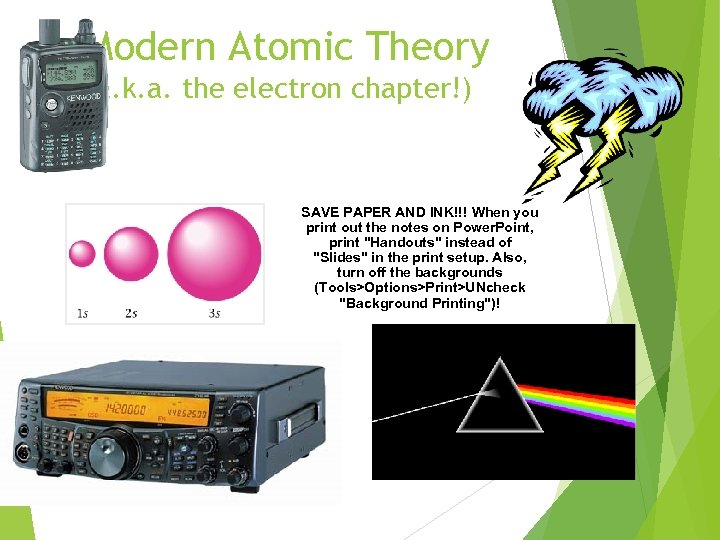Modern Atomic Theory (a. k. a. the electron chapter!) SAVE PAPER AND INK!!! When you print out the notes on Power. Point, print "Handouts" instead of "Slides" in the print setup. Also, turn off the backgrounds (Tools>Options>Print>UNcheck "Background Printing")!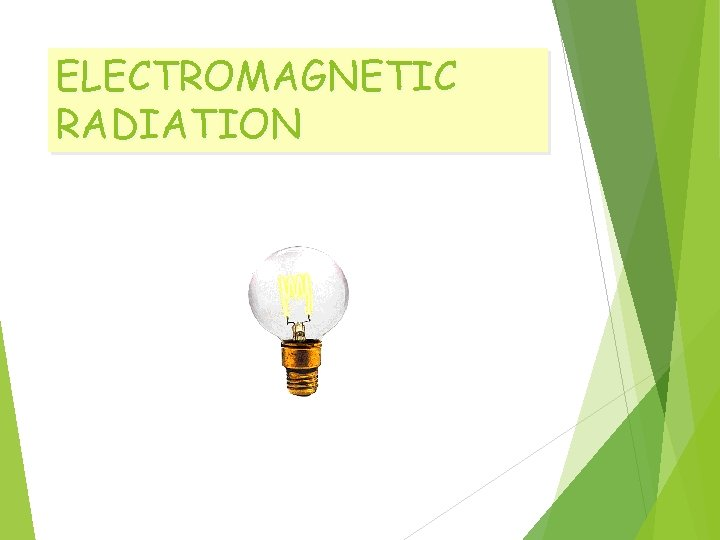ELECTROMAGNETIC RADIATION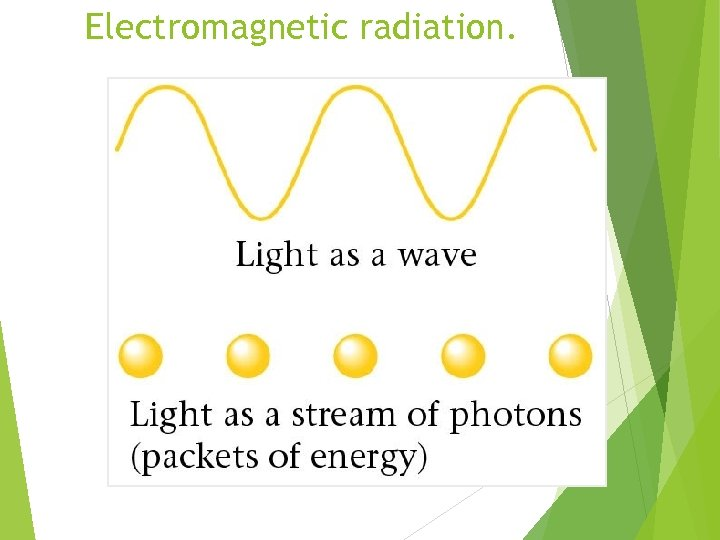Electromagnetic radiation.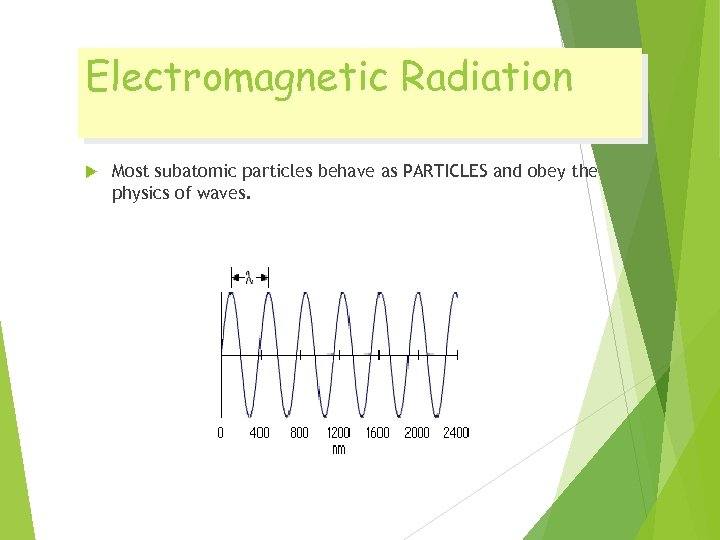Electromagnetic Radiation Most subatomic particles behave as PARTICLES and obey the physics of waves.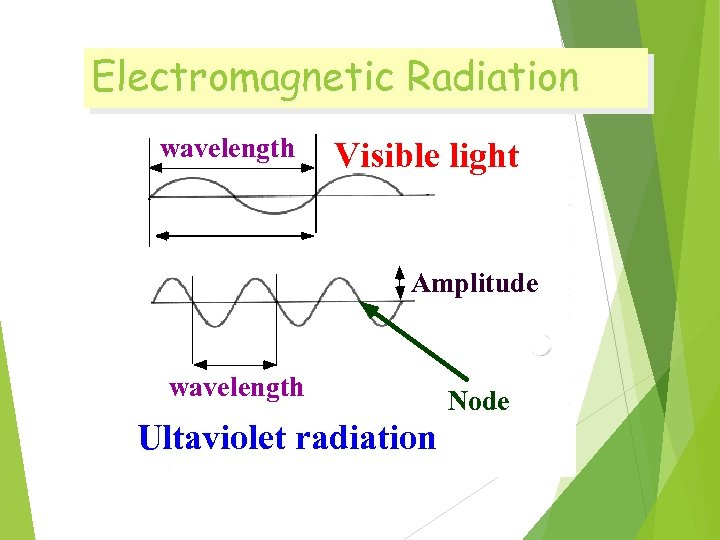Electromagnetic Radiation wavelength Visible light Amplitude wavelength Ultaviolet radiation Node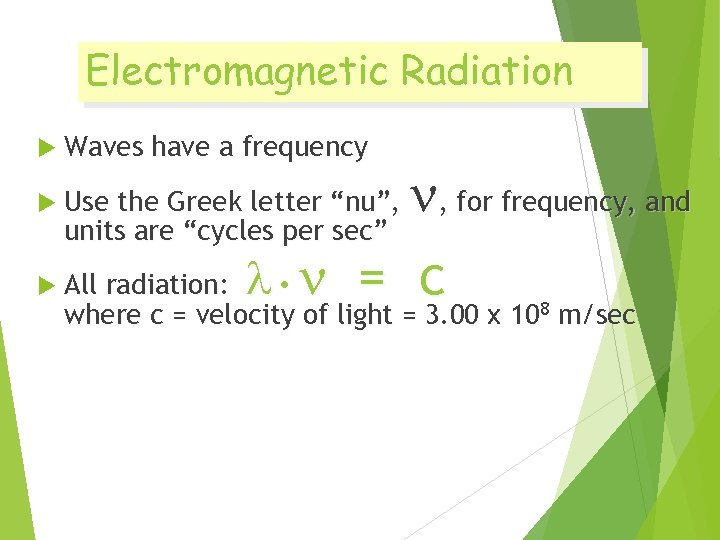Electromagnetic Radiation Waves have a frequency , for frequency, and All radiation: • = c Use the Greek letter “nu”, units are “cycles per sec” where c = velocity of light = 3. 00 x 108 m/sec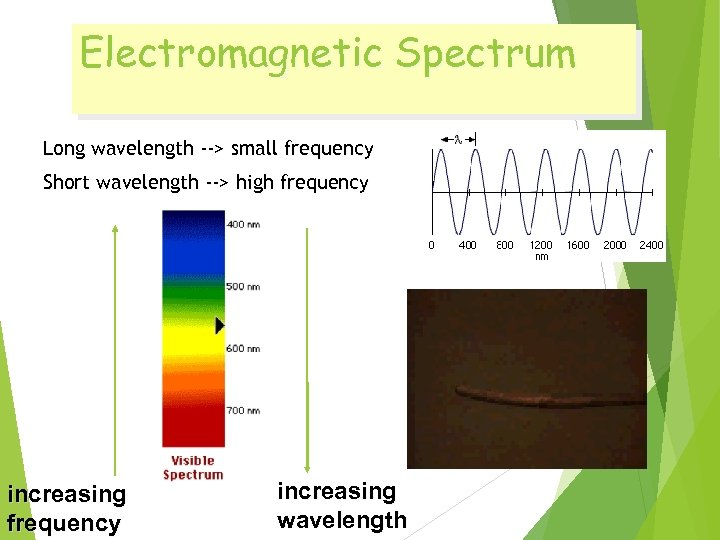Electromagnetic Spectrum Long wavelength --> small frequency Short wavelength --> high frequency increasing wavelength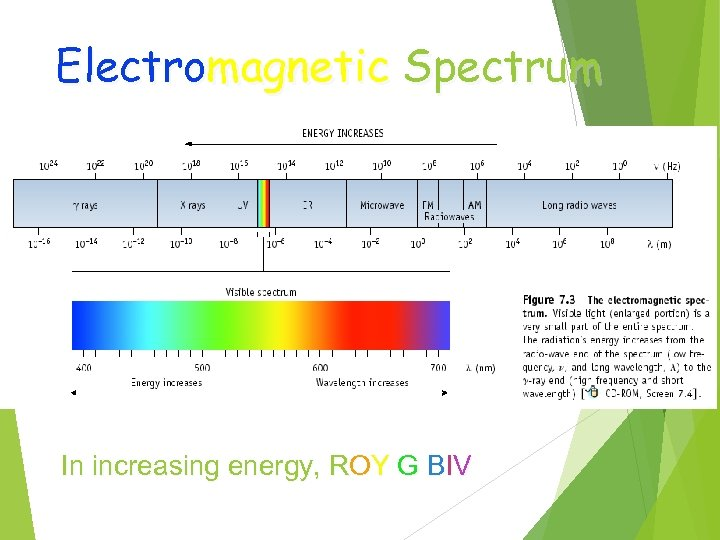Electromagnetic Spectrum In increasing energy, ROY G BIVExcited Gases & Atomic Structure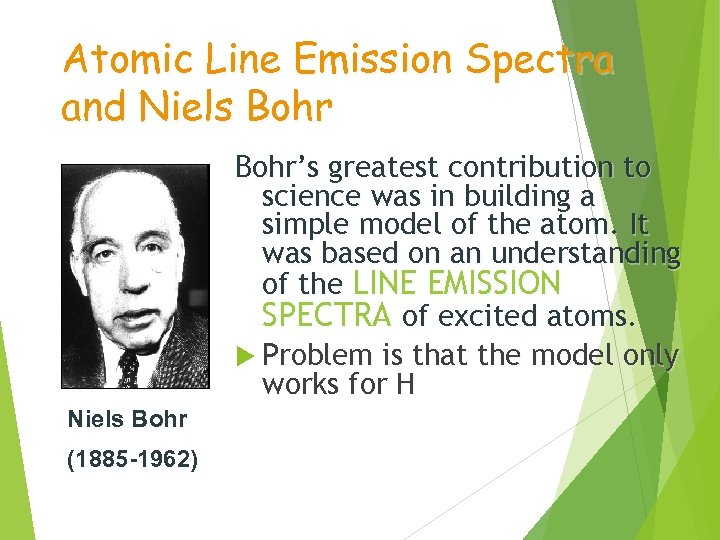Atomic Line Emission Spectra and Niels Bohr’s greatest contribution to science was in building a simple model of the atom. It was based on an understanding of the LINE EMISSION SPECTRA of excited atoms. Problem is that the model only works for H Niels Bohr (1885 -1962)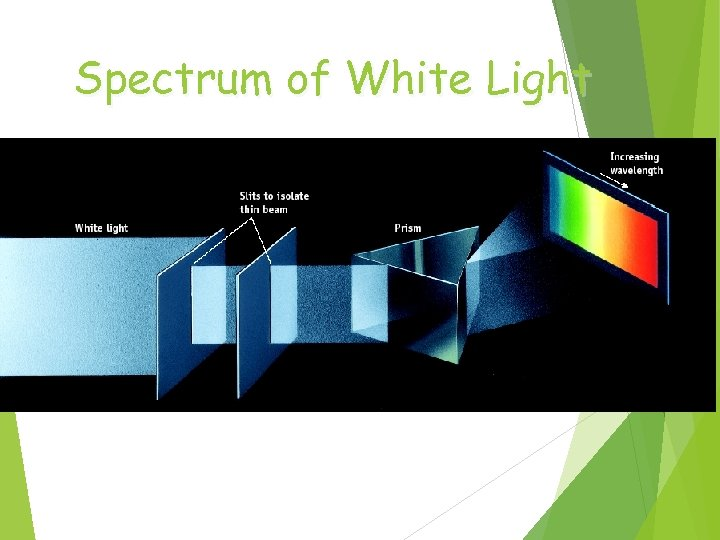Spectrum of White Light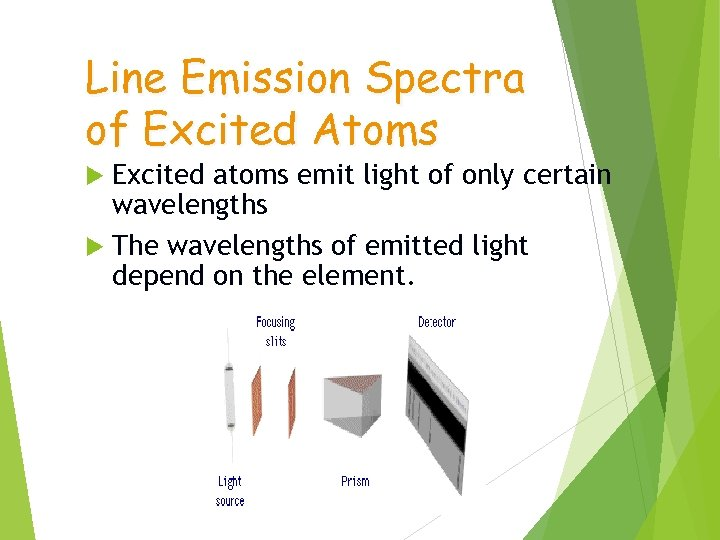Line Emission Spectra of Excited Atoms Excited atoms emit light of only certain wavelengths The wavelengths of emitted light depend on the element.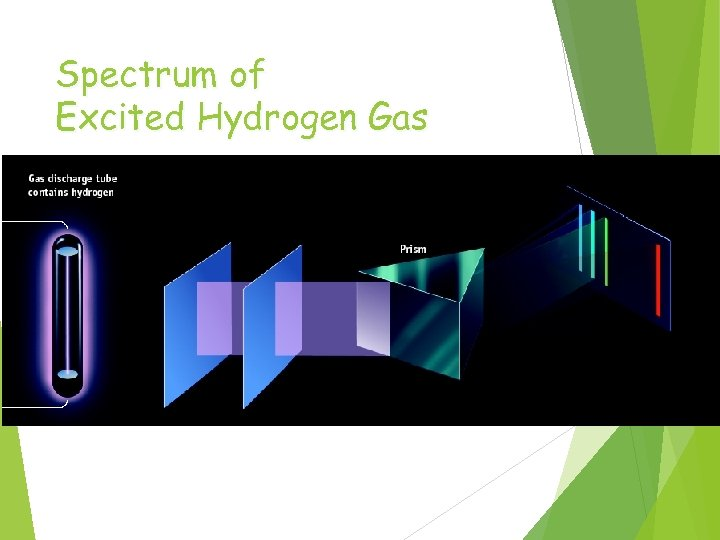Spectrum of Excited Hydrogen Gas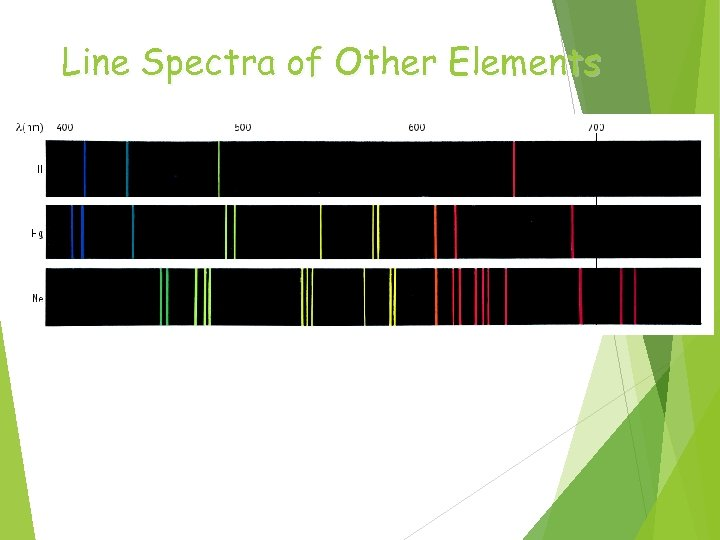Line Spectra of Other Elements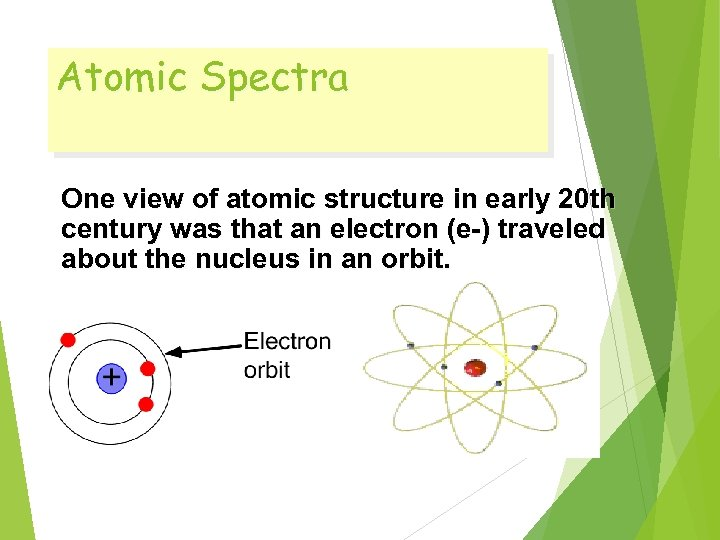Atomic Spectra One view of atomic structure in early 20 th century was that an electron (e-) traveled about the nucleus in an orbit.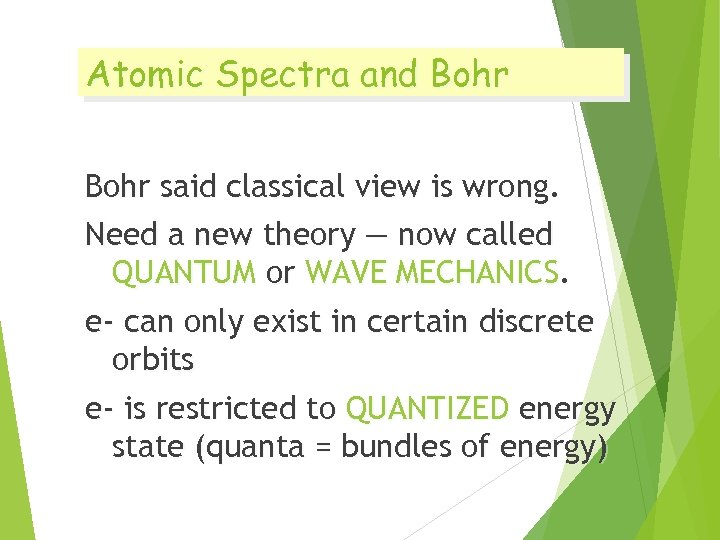Atomic Spectra and Bohr said classical view is wrong. Need a new theory — now called QUANTUM or WAVE MECHANICS. e- can only exist in certain discrete orbits e- is restricted to QUANTIZED energy state (quanta = bundles of energy)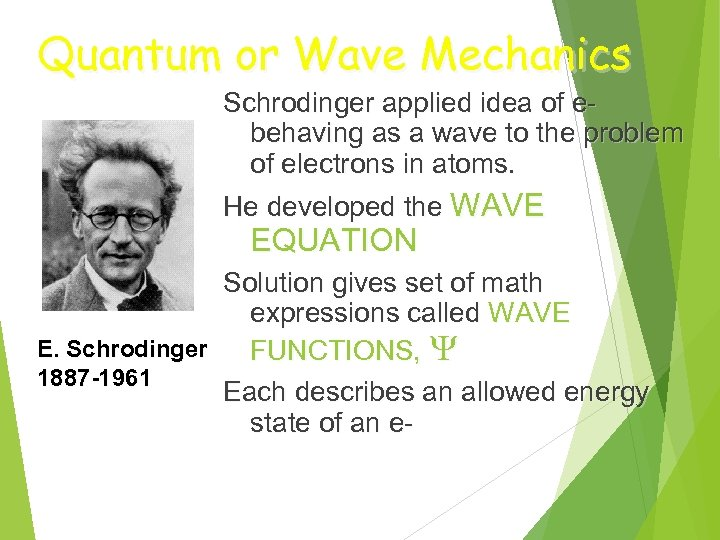Quantum or Wave Mechanics Schrodinger applied idea of ebehaving as a wave to the problem of electrons in atoms. He developed the WAVE EQUATION Solution gives set of math expressions called WAVE E. Schrodinger FUNCTIONS, 1887 -1961 Each describes an allowed energy state of an e-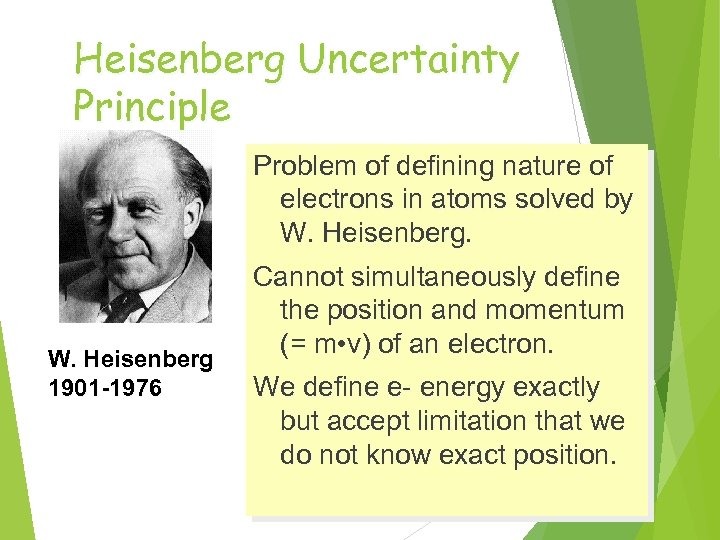Heisenberg Uncertainty Principle Problem of defining nature of electrons in atoms solved by W. Heisenberg 1901 -1976 Cannot simultaneously define the position and momentum (= m • v) of an electron. We define e- energy exactly but accept limitation that we do not know exact position.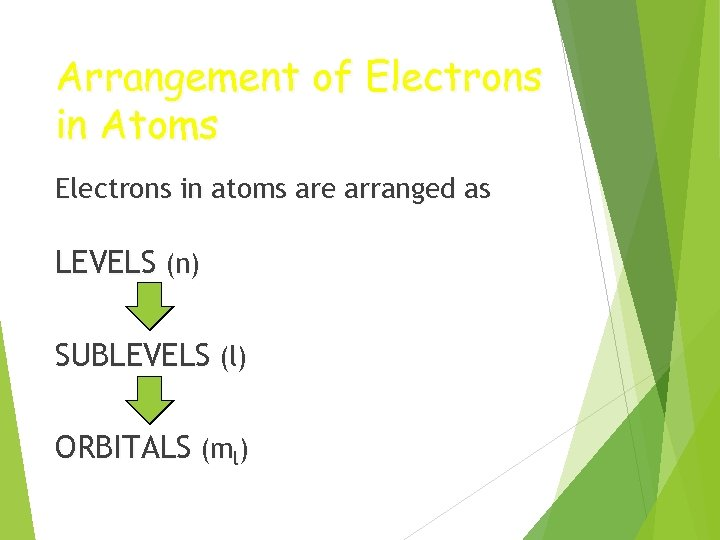Arrangement of Electrons in Atoms Electrons in atoms are arranged as LEVELS (n) SUBLEVELS (l) ORBITALS (ml)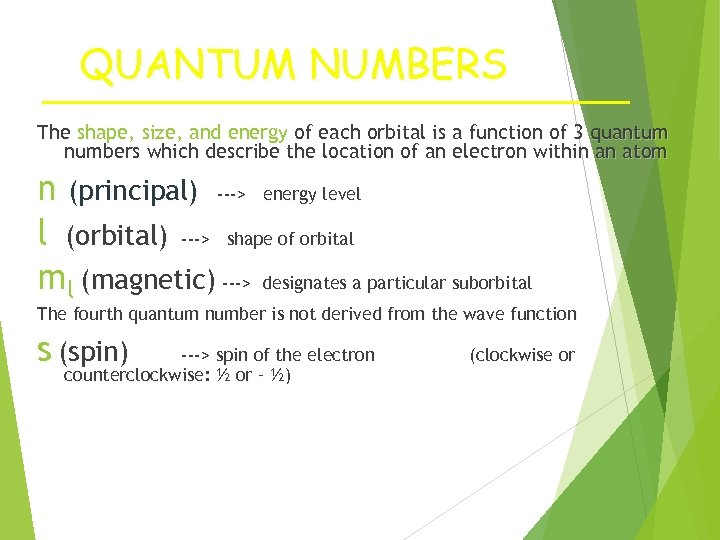QUANTUM NUMBERS The shape, size, and energy of each orbital is a function of 3 quantum numbers which describe the location of an electron within an atom n (principal) ---> energy level l (orbital) ---> shape of orbital ml (magnetic) ---> designates a particular suborbital The fourth quantum number is not derived from the wave function s (spin) ---> spin of the electron counterclockwise: ½ or – ½) (clockwise or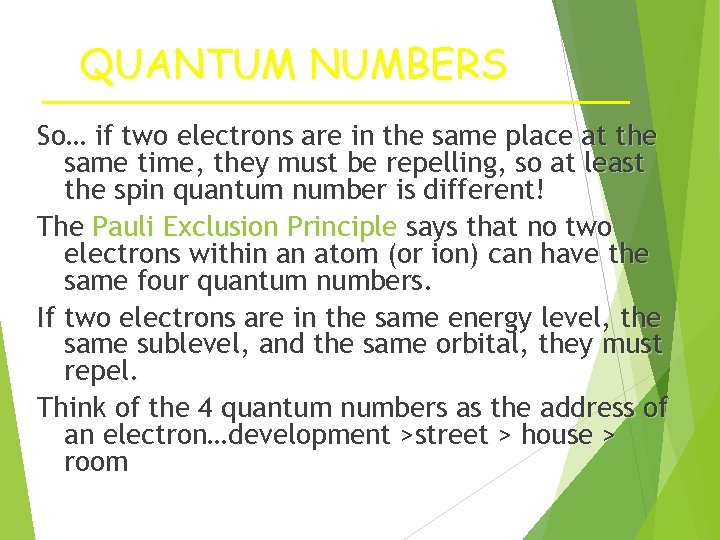QUANTUM NUMBERS So… if two electrons are in the same place at the same time, they must be repelling, so at least the spin quantum number is different! The Pauli Exclusion Principle says that no two electrons within an atom (or ion) can have the same four quantum numbers. If two electrons are in the same energy level, the same sublevel, and the same orbital, they must repel. Think of the 4 quantum numbers as the address of an electron…development >street > house > room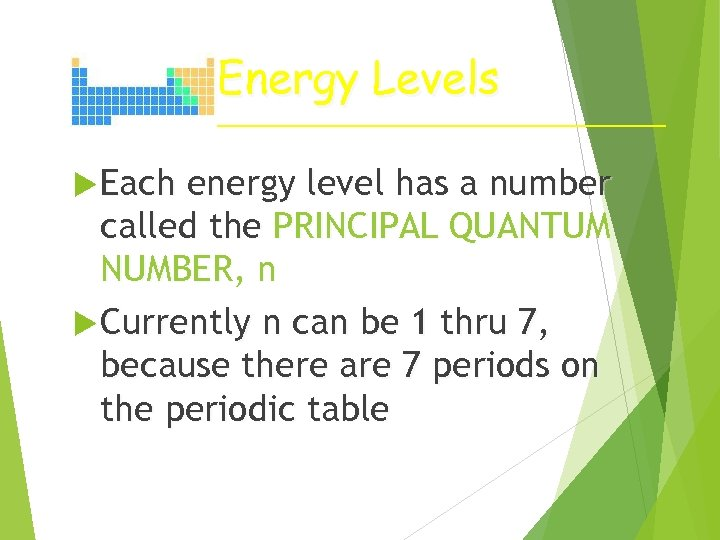Energy Levels Each energy level has a number called the PRINCIPAL QUANTUM NUMBER, n Currently n can be 1 thru 7, because there are 7 periods on the periodic table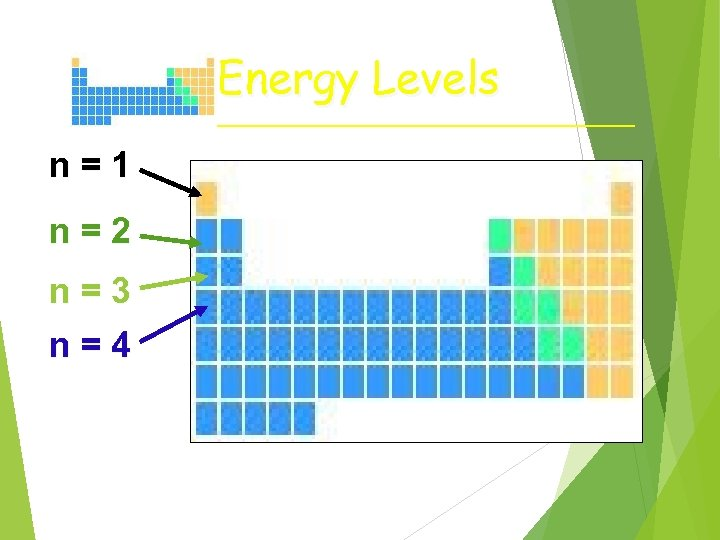Energy Levels n=1 n=2 n=3 n=4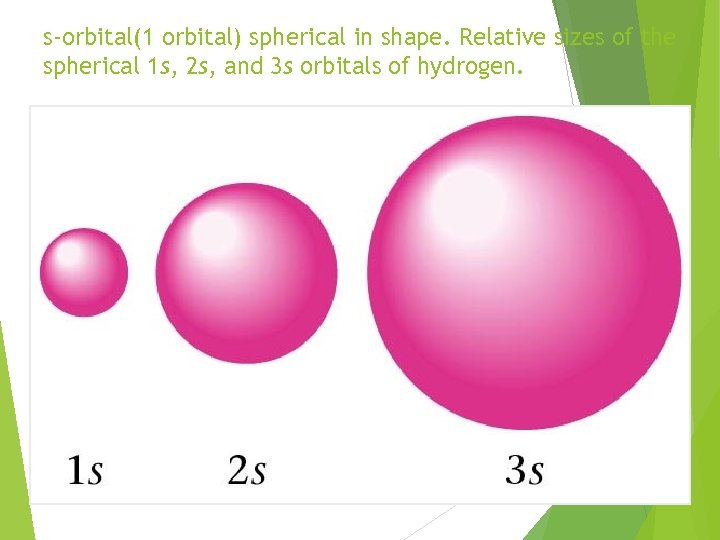s-orbital(1 orbital) spherical in shape. Relative sizes of the spherical 1 s, 2 s, and 3 s orbitals of hydrogen.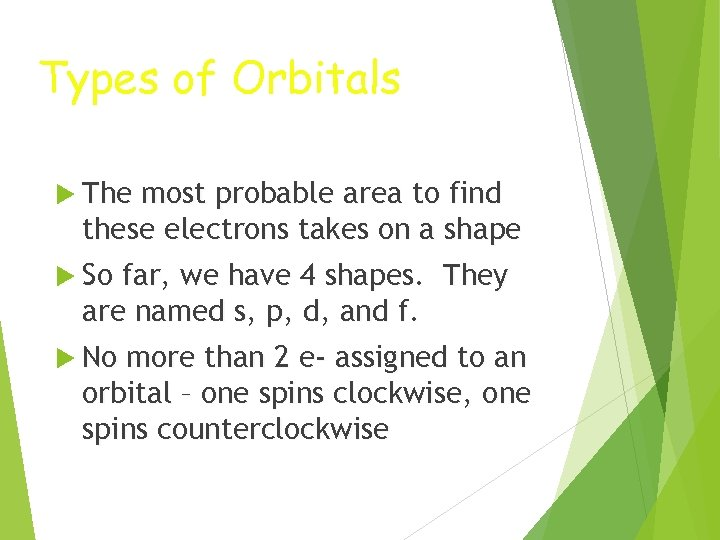Types of Orbitals The most probable area to find these electrons takes on a shape So far, we have 4 shapes. They are named s, p, d, and f. No more than 2 e- assigned to an orbital – one spins clockwise, one spins counterclockwise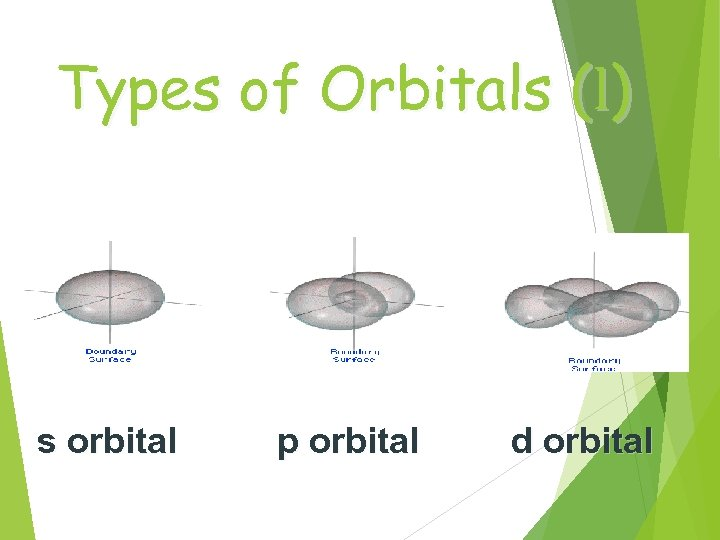Types of Orbitals (l) s orbital p orbital d orbital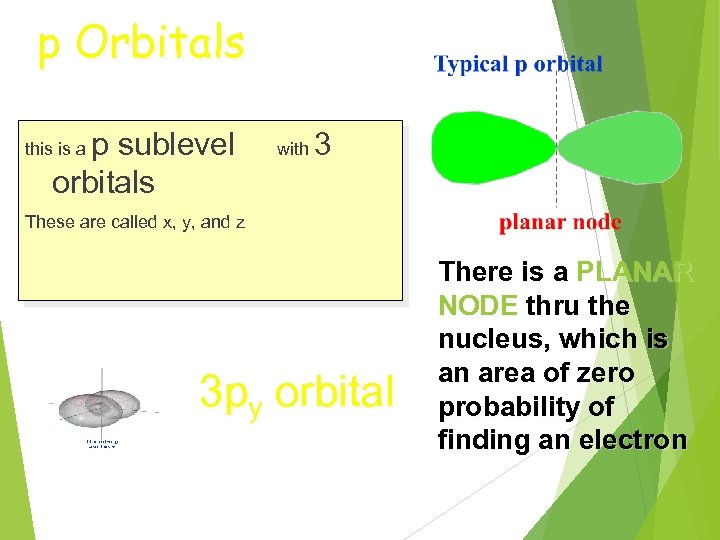p Orbitals p sublevel orbitals this is a with 3 These are called x, y, and z 3 py orbital There is a PLANAR NODE thru the nucleus, which is an area of zero probability of finding an electron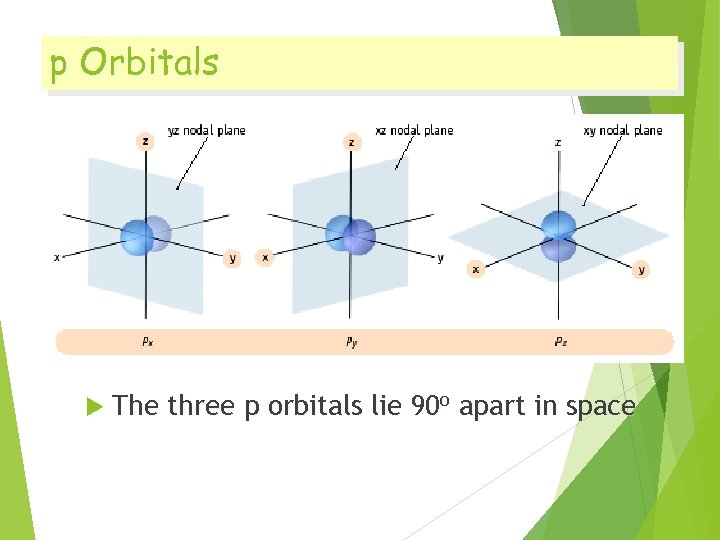p Orbitals The three p orbitals lie 90 o apart in space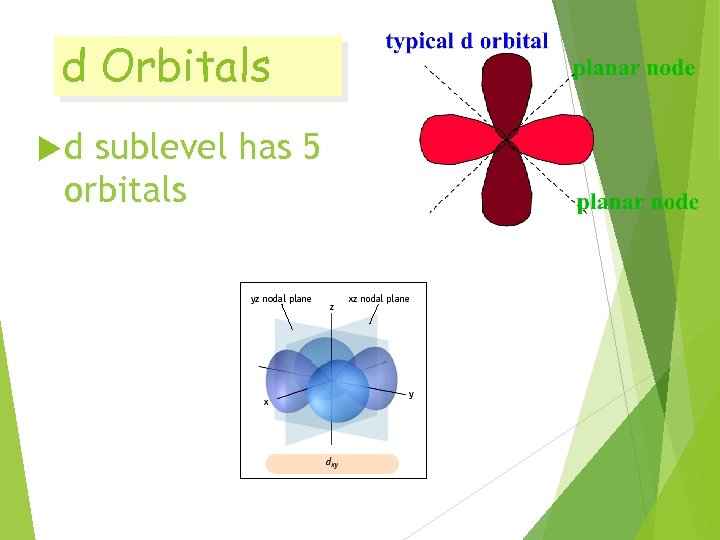d Orbitals d sublevel has 5 orbitals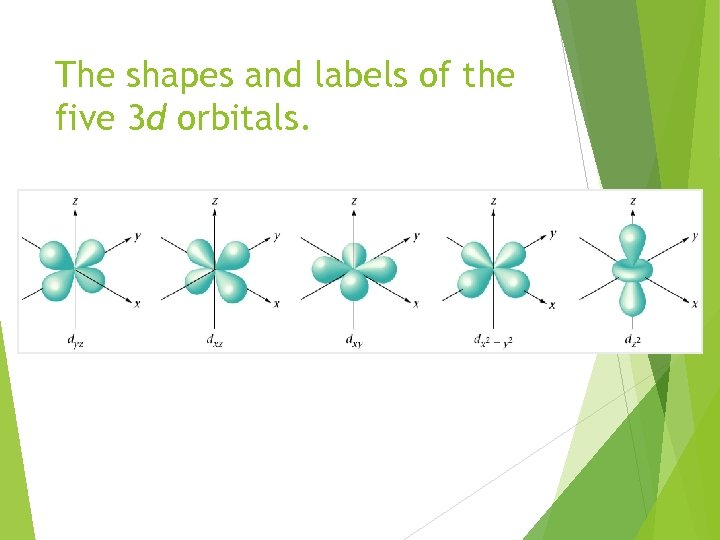The shapes and labels of the five 3 d orbitals.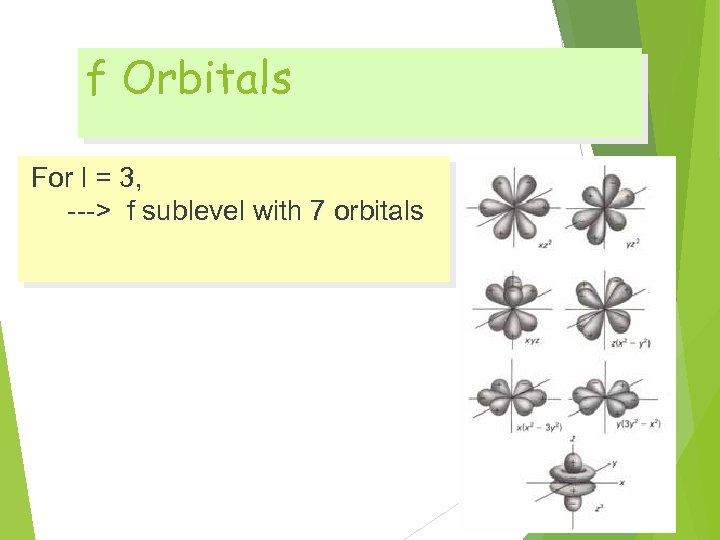f Orbitals For l = 3, ---> f sublevel with 7 orbitals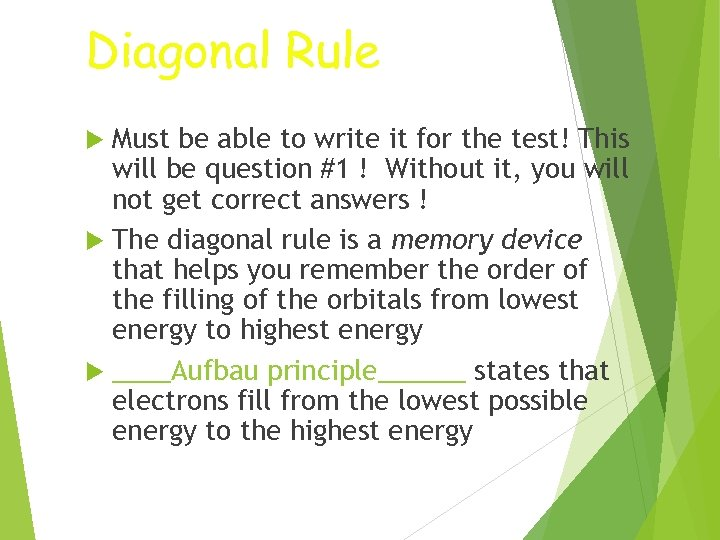Diagonal Rule Must be able to write it for the test! This will be question #1 ! Without it, you will not get correct answers ! The diagonal rule is a memory device that helps you remember the order of the filling of the orbitals from lowest energy to highest energy ____Aufbau principle______ states that electrons fill from the lowest possible energy to the highest energy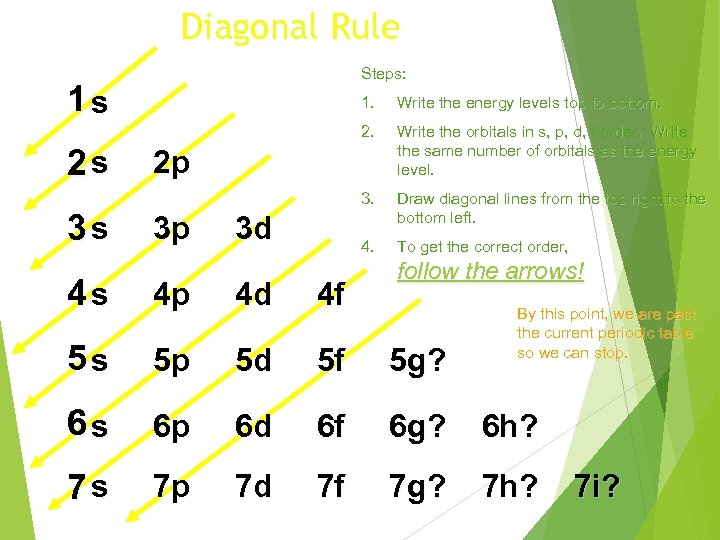Diagonal Rule Steps: 1 s 3 s Write the energy levels top to bottom. 2 s 1. Write the orbitals in s, p, d, f order. Write the same number of orbitals as the energy level. 3. Draw diagonal lines from the top right to the bottom left. 4. To get the correct order, 2 p 3 p 3 d follow the arrows! 4 s 4 p 4 d 4 f 5 s 5 p 5 d 5 f 5 g? 6 s 6 p 6 d 6 f 6 g? 6 h? 7 s 7 p 7 d 7 f 7 g? 7 h? By this point, we are past the current periodic table so we can stop. 7 i?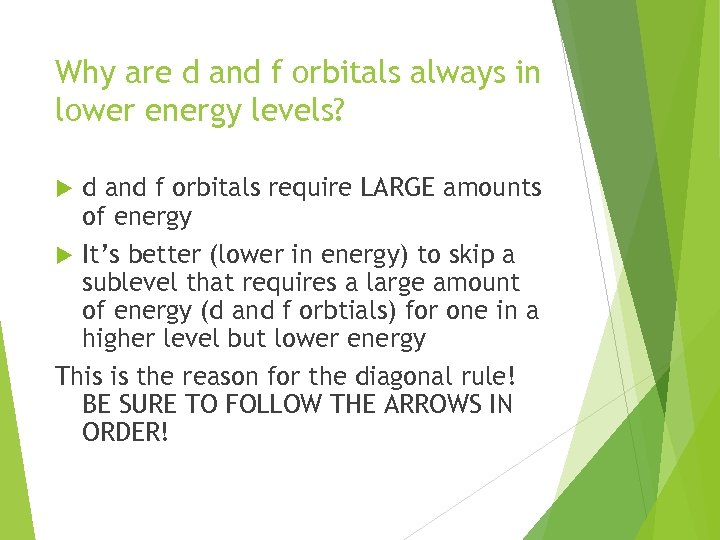Why are d and f orbitals always in lower energy levels? d and f orbitals require LARGE amounts of energy It’s better (lower in energy) to skip a sublevel that requires a large amount of energy (d and f orbtials) for one in a higher level but lower energy This is the reason for the diagonal rule! BE SURE TO FOLLOW THE ARROWS IN ORDER!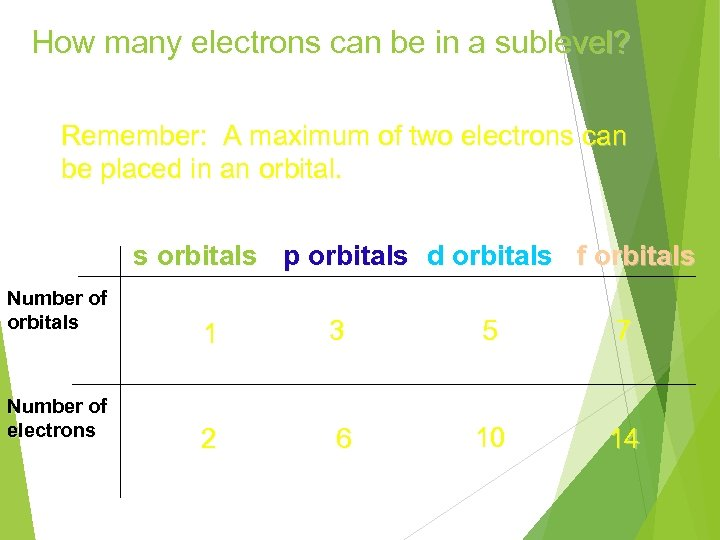How many electrons can be in a sublevel? Remember: A maximum of two electrons can be placed in an orbital. s orbitals p orbitals d orbitals f orbitals Number of electrons 1 3 5 7 2 6 10 14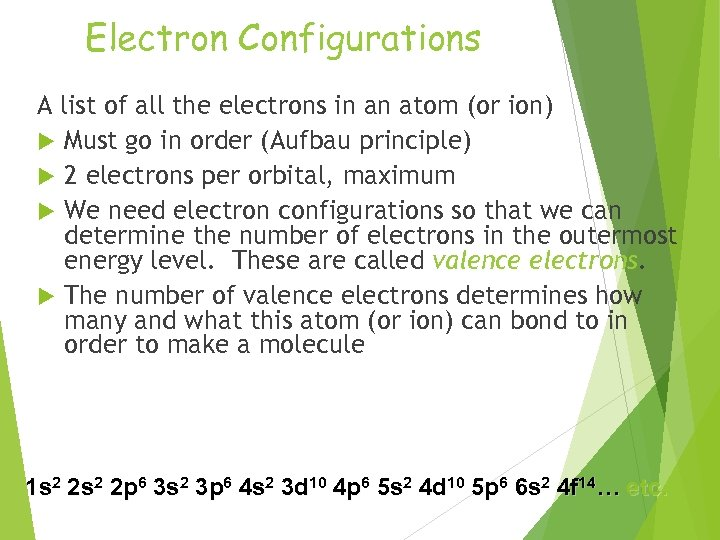Electron Configurations A list of all the electrons in an atom (or ion) Must go in order (Aufbau principle) 2 electrons per orbital, maximum We need electron configurations so that we can determine the number of electrons in the outermost energy level. These are called valence electrons. The number of valence electrons determines how many and what this atom (or ion) can bond to in order to make a molecule 1 s 2 2 p 6 3 s 2 3 p 6 4 s 2 3 d 10 4 p 6 5 s 2 4 d 10 5 p 6 6 s 2 4 f 14… etc.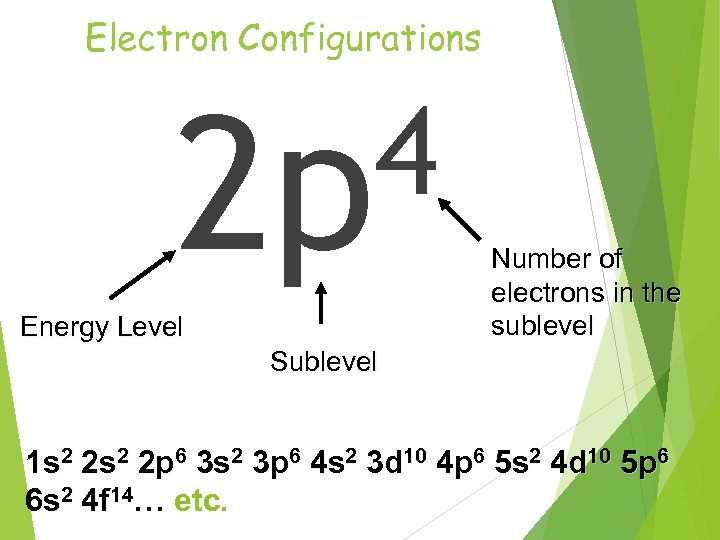Electron Configurations 4 2 p Energy Level Number of electrons in the sublevel Sublevel 1 s 2 2 p 6 3 s 2 3 p 6 4 s 2 3 d 10 4 p 6 5 s 2 4 d 10 5 p 6 6 s 2 4 f 14… etc.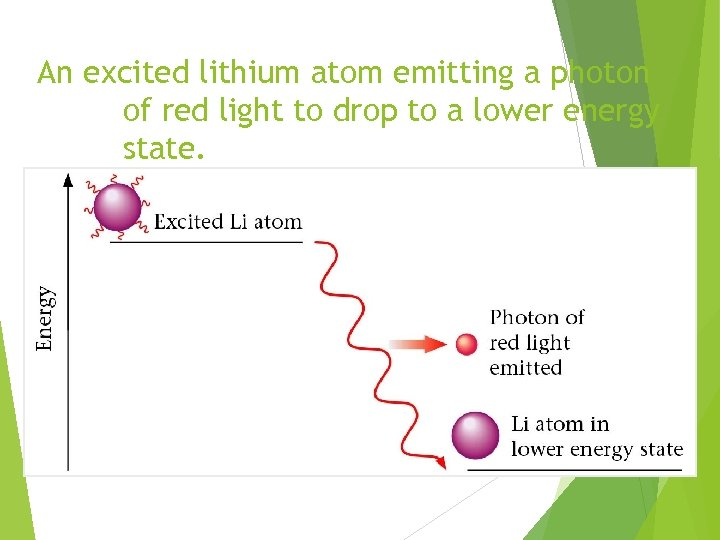An excited lithium atom emitting a photon of red light to drop to a lower energy state.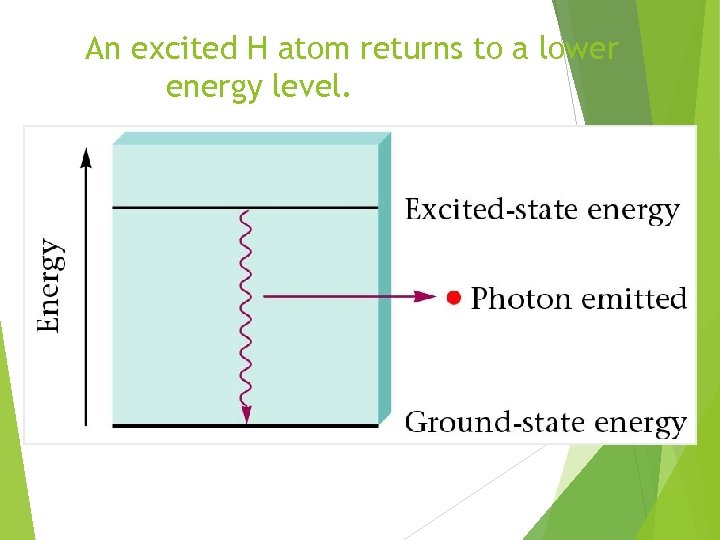An excited H atom returns to a lower energy level.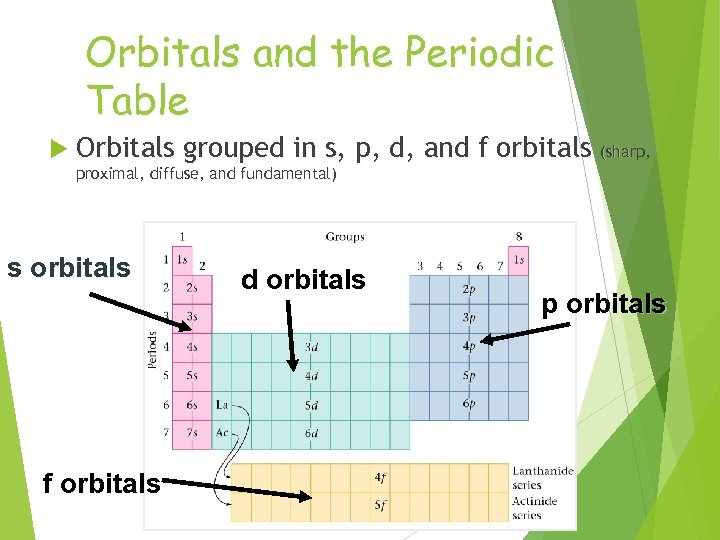Orbitals and the Periodic Table Orbitals grouped in s, p, d, and f orbitals (sharp, proximal, diffuse, and fundamental) s orbitals f orbitals d orbitals p orbitals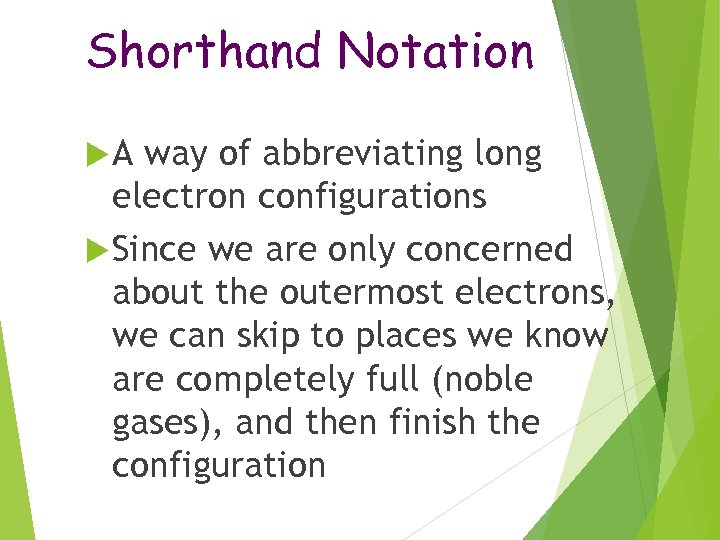Shorthand Notation A way of abbreviating long electron configurations Since we are only concerned about the outermost electrons, we can skip to places we know are completely full (noble gases), and then finish the configuration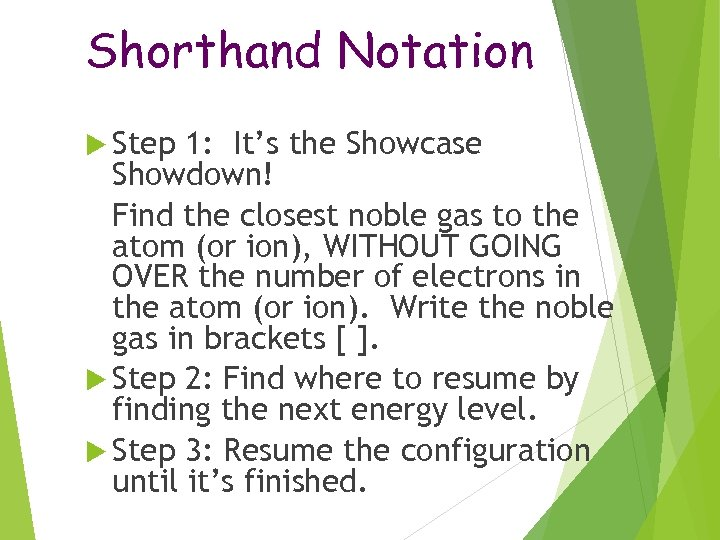Shorthand Notation Step 1: It’s the Showcase Showdown! Find the closest noble gas to the atom (or ion), WITHOUT GOING OVER the number of electrons in the atom (or ion). Write the noble gas in brackets [ ]. Step 2: Find where to resume by finding the next energy level. Step 3: Resume the configuration until it’s finished.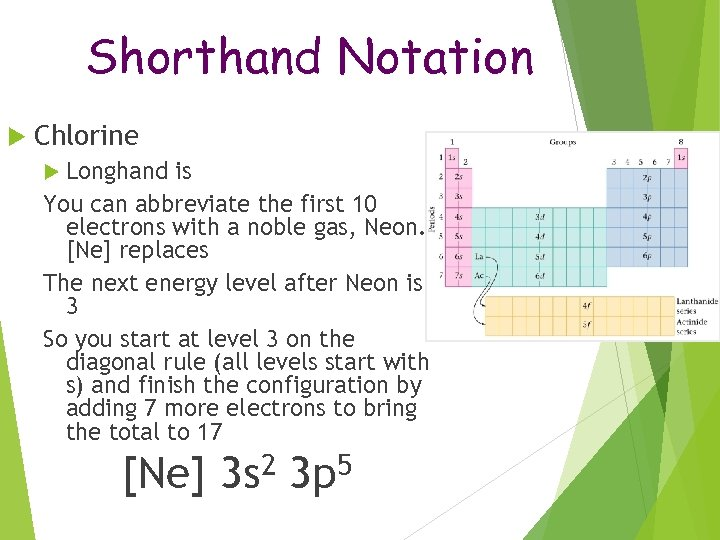Shorthand Notation Chlorine Longhand is 1 s 2 2 p 6 3 s 2 3 p 5 You can abbreviate the first 10 electrons with a noble gas, Neon. [Ne] replaces 1 s 2 2 p 6 The next energy level after Neon is 3 So you start at level 3 on the diagonal rule (all levels start with s) and finish the configuration by adding 7 more electrons to bring the total to 17 [Ne] 2 3 s 5 3 p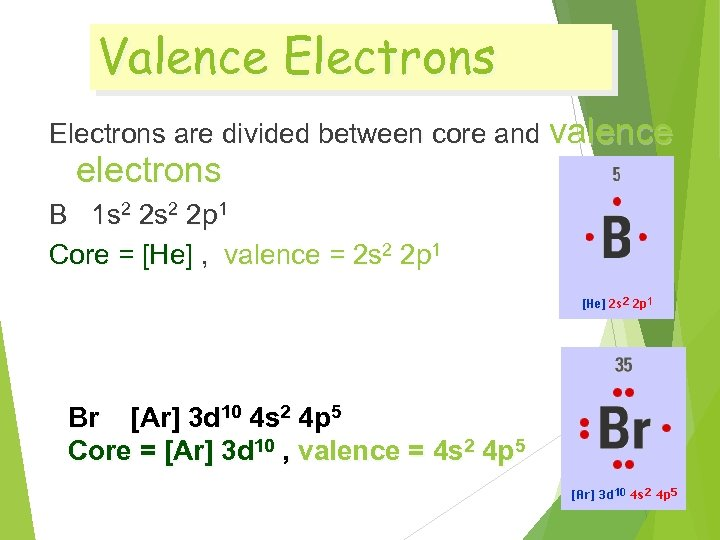Valence Electrons are divided between core and valence electrons B 1 s 2 2 p 1 Core = [He] , valence = 2 s 2 2 p 1 Br [Ar] 3 d 10 4 s 2 4 p 5 Core = [Ar] 3 d 10 , valence = 4 s 2 4 p 5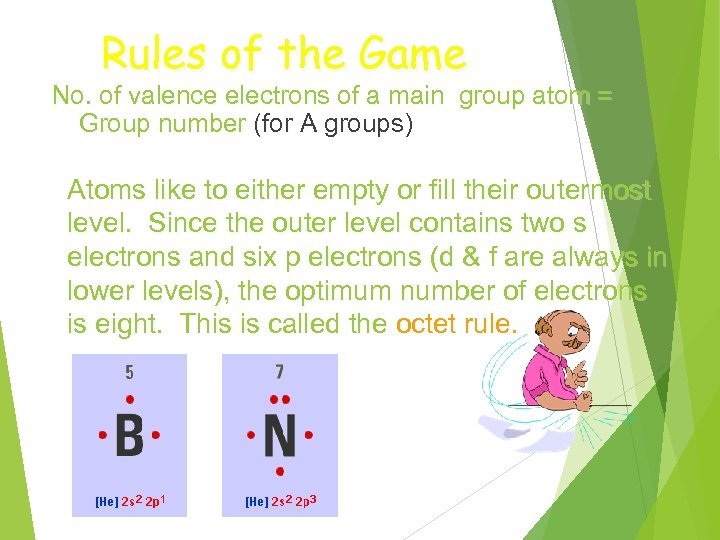Rules of the Game No. of valence electrons of a main group atom = Group number (for A groups) Atoms like to either empty or fill their outermost level. Since the outer level contains two s electrons and six p electrons (d & f are always in lower levels), the optimum number of electrons is eight. This is called the octet rule.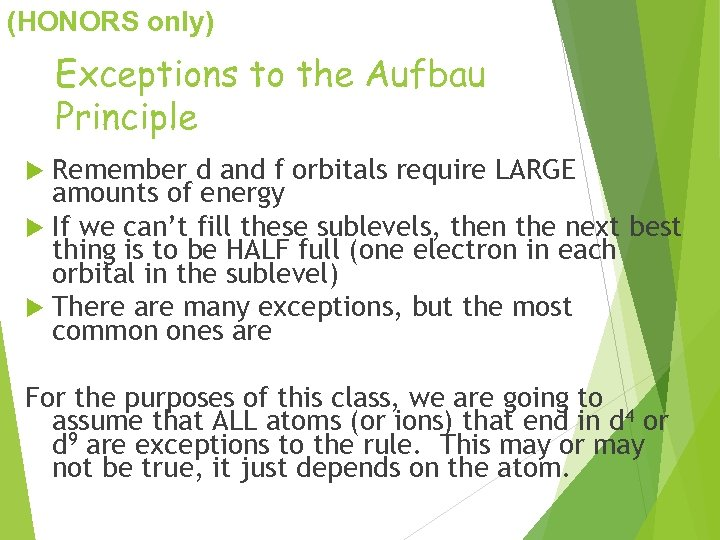(HONORS only) Exceptions to the Aufbau Principle Remember d and f orbitals require LARGE amounts of energy If we can’t fill these sublevels, then the next best thing is to be HALF full (one electron in each orbital in the sublevel) There are many exceptions, but the most common ones are d 4 and d 9 For the purposes of this class, we are going to assume that ALL atoms (or ions) that end in d 4 or d 9 are exceptions to the rule. This may or may not be true, it just depends on the atom.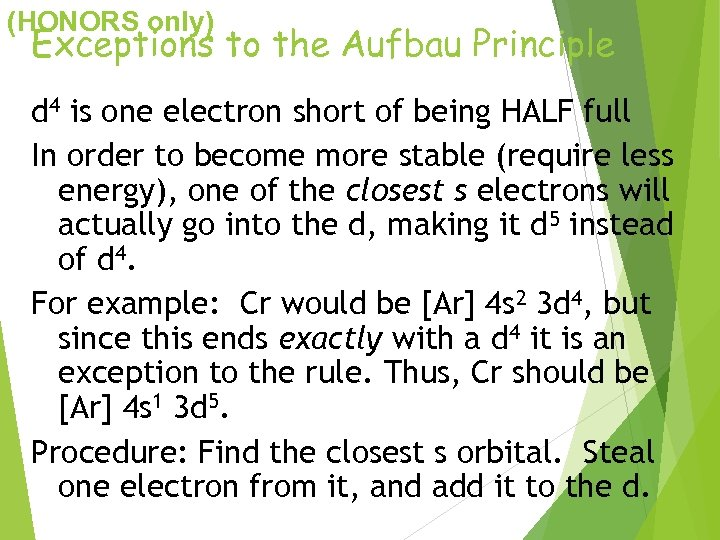(HONORS only) Exceptions to the Aufbau Principle d 4 is one electron short of being HALF full In order to become more stable (require less energy), one of the closest s electrons will actually go into the d, making it d 5 instead of d 4. For example: Cr would be [Ar] 4 s 2 3 d 4, but since this ends exactly with a d 4 it is an exception to the rule. Thus, Cr should be [Ar] 4 s 1 3 d 5. Procedure: Find the closest s orbital. Steal one electron from it, and add it to the d.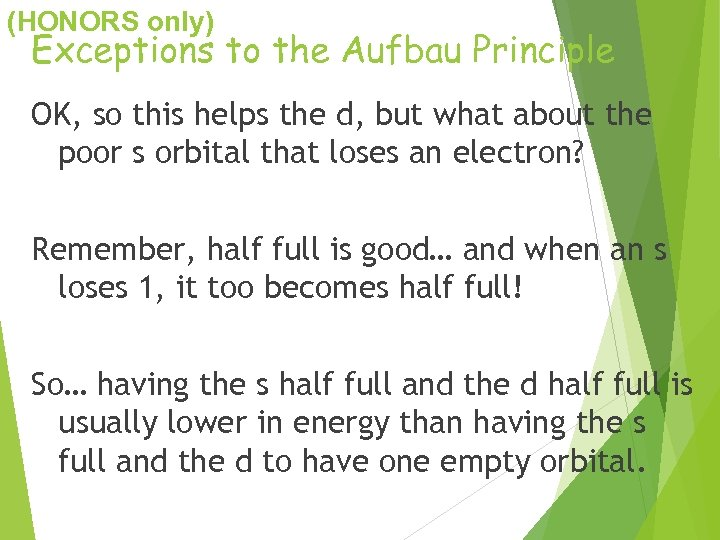(HONORS only) Exceptions to the Aufbau Principle OK, so this helps the d, but what about the poor s orbital that loses an electron? Remember, half full is good… and when an s loses 1, it too becomes half full! So… having the s half full and the d half full is usually lower in energy than having the s full and the d to have one empty orbital.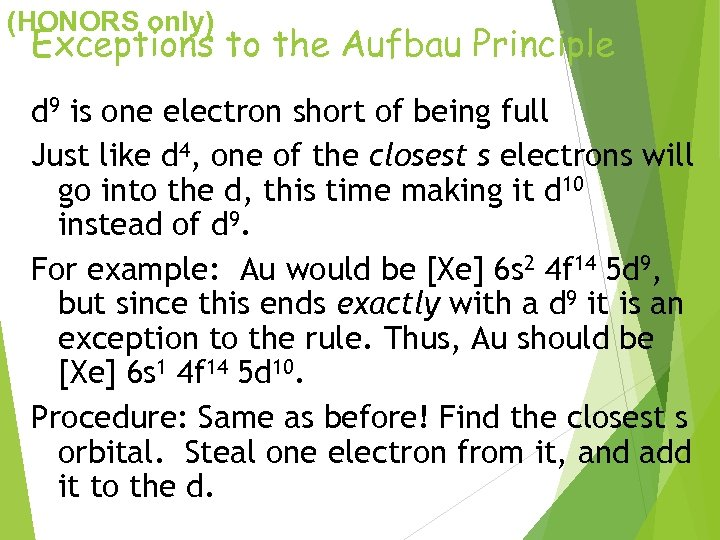(HONORS only) Exceptions to the Aufbau Principle d 9 is one electron short of being full Just like d 4, one of the closest s electrons will go into the d, this time making it d 10 instead of d 9. For example: Au would be [Xe] 6 s 2 4 f 14 5 d 9, but since this ends exactly with a d 9 it is an exception to the rule. Thus, Au should be [Xe] 6 s 1 4 f 14 5 d 10. Procedure: Same as before! Find the closest s orbital. Steal one electron from it, and add it to the d.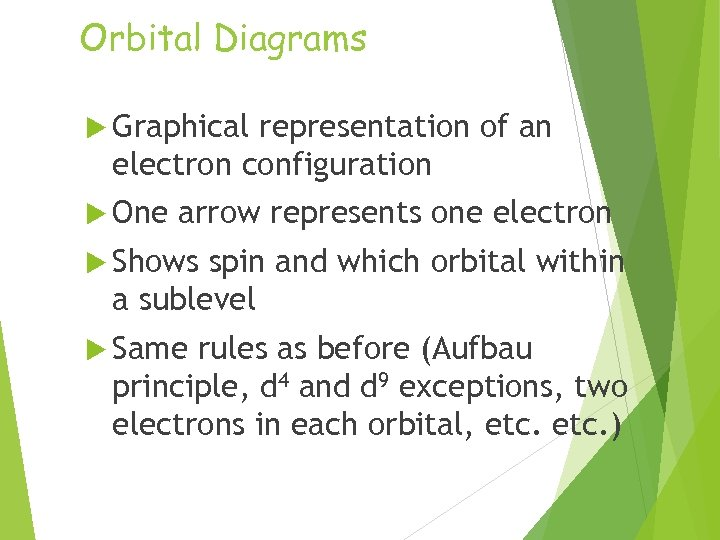Orbital Diagrams Graphical representation of an electron configuration One arrow represents one electron Shows spin and which orbital within a sublevel Same rules as before (Aufbau principle, d 4 and d 9 exceptions, two electrons in each orbital, etc. )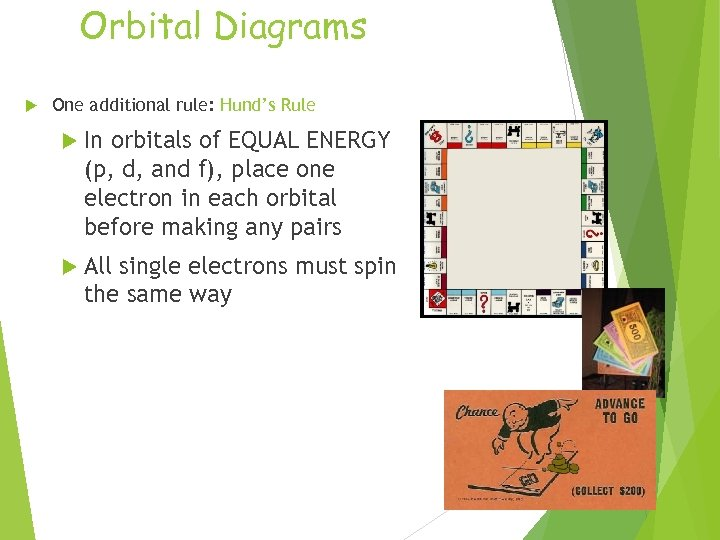Orbital Diagrams One additional rule: Hund’s Rule In orbitals of EQUAL ENERGY (p, d, and f), place one electron in each orbital before making any pairs All single electrons must spin the same way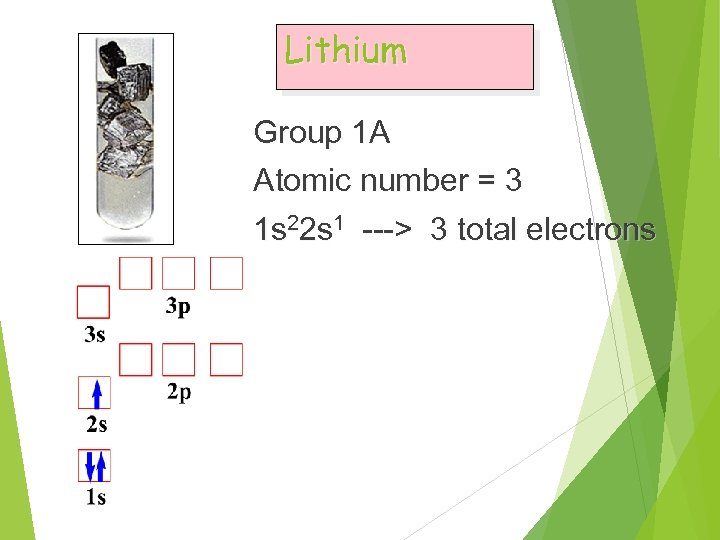Lithium Group 1 A Atomic number = 3 1 s 22 s 1 ---> 3 total electrons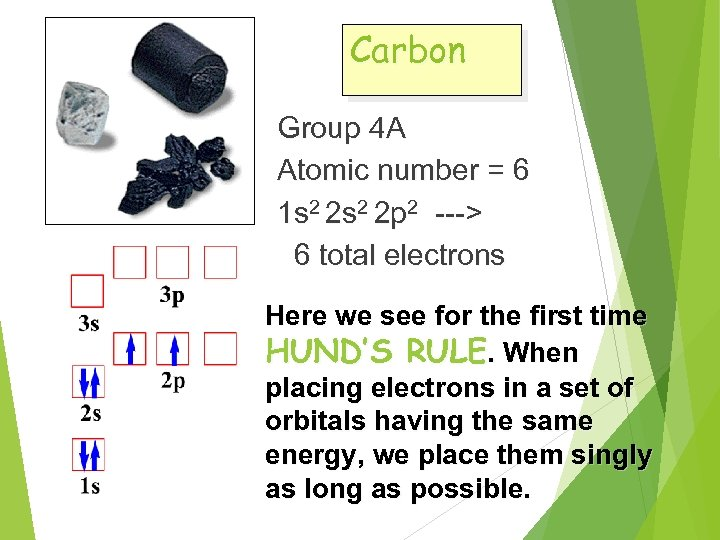Carbon Group 4 A Atomic number = 6 1 s 2 2 p 2 ---> 6 total electrons Here we see for the first time HUND’S RULE. When placing electrons in a set of orbitals having the same energy, we place them singly as long as possible.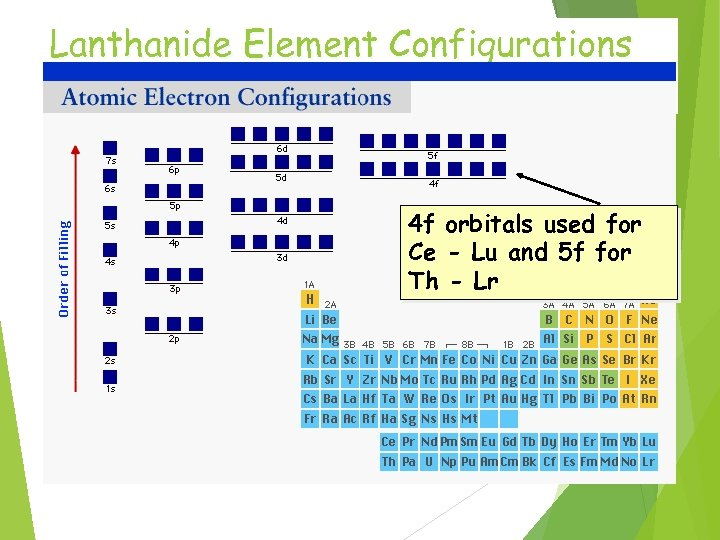Lanthanide Element Configurations 4 f orbitals used for Ce - Lu and 5 f for Th - Lr